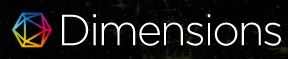### MODEL REGRESI LINIER BAYESIAN DENGAN APLIKASI PADA DATA PENUNDAAN PENERBANGAN

Vemmie Nastiti Lestari, Subanar Subanar

DOI: https://doi.org/10.14710/jfma.v1i2.21

#### Abstract

Bayesian linear regression is an approach to linear regression where statistical analysis depend of Bayesian inference. The Bayesian model on big data uses a summary of data statistics as input; Statistical summary can be calculated from each subset, then a statistical summary of the full dataset is obtained from the sum of the summary statistics for each subset. Recent developments in data science and research, produce large datasets that are too large to be analyzed as a whole due to the limitations of computer memory or storage capacity. To overcome this, a program package was introduced from R namely BayesSummaryStatLM for the Bayesian linear regression model with the Markov Chain Monte Carlo implementation that overcomes this limitation. Then the program package from R, ff is used to read data in large datasets while calculating statistics summary. In this study Bayesian linear regression model used with several choices of prior distribution for unknown model parameters, and illustrates in simulation data and real datasets for flight delay data in US 2008. The application of simulation data and flight delay data produces a plot of density functions for the β parameters has a shape resembling a plot of Normal distribution density function, whereas for plot  parameters the density function has a shape resembling the plot of Inverse Gamma distribution density function. In the simulation data, the estimator for each parameter produced has a value that approach to the value of the specified parameter (True Value). This is also indicated by the narrow credible interval for each parameters.

PDF

#### References

R Core Team, "R: A Languange and Environment for Statistical Computing," The R Project for Statistical Computing website, 2014. [Online]. Available: http://www.R-project.org/. [Accessed 11 March 2017].

Adler D, Glaser C, Nenadic O, Oehlschlagel J, Z, "ff: memory-efficient storage of large data on disk and fast access functions," R package version 2.2-13, 2013. [Online]. Available: http://CRAN.R-project.org/package=ff. [Accessed 10 March 2017].

Ordonez C, Garcia-Alvarado C, Baladandayuthapan, "Bayesian variable selection in linear regression in one pass for large data sets," ACM Transactions on Knowledge Discovery from Data, vol. 9(1), no. 3, p. doi: 10.1145/26296178, 2014.

Ghosh J, Reiter JP, "Secure Bayesian model averaging for horizontally partitioned data," Statistics and Computing, vol. 23, pp. 311-322, 2013.

Carlin BP, Louis TA, Bayesian Methods for Data Analysis. 3rd ed, Boca Raton: FL: Chapman and Hall/CRC Press, 2009.

Gelman A, Carlin JB, Stern HS, Dunson DB, Vehta, Bayesian Data Analysis 3rd ed, Boca Raton: FL: Chapman and Hall/CRC Press, 2013.

Alexey M, Evgeny S, Erin M C, "BayesSummaryStatLM: An R package for Bayesian Linear Models for Big data and Data Science".

United States Department of Transportation, "Bureau of Transportation Statistics," [Online]. Available: http://stat-computing.org/dataexpo/2009/the-data.html. [Accessed 25 September 2017].

Evgeny Savel'ev, Alexey Miroshnikov, Erin Conlon, "MCMC Sampling of Bayesian Linear Models via Summary Statistics", 2015.

Robert CP, Casella G, Monte Carlo Statistical Methods, 2nd ed, New York, NY:

Springer, 2004.

Lindley DV, Smith AFM, "Bayes estimates for the linear model", J R Stat Soc B,

, vol 34, pp. 1-41.

### Refbacks

• There are currently no refbacks.

### Publisher:

Department of Mathematics, Faculty of Science and Mathematics, Diponegoro University

Mailing address: Jl. Prof Soedarto, SH, Tembalang, Semarang, Indonesia 50275

Telp./Fax             : (+6224) 70789493 / (+62224) 76480922

Website              : www.math.fsm.undip.ac.id